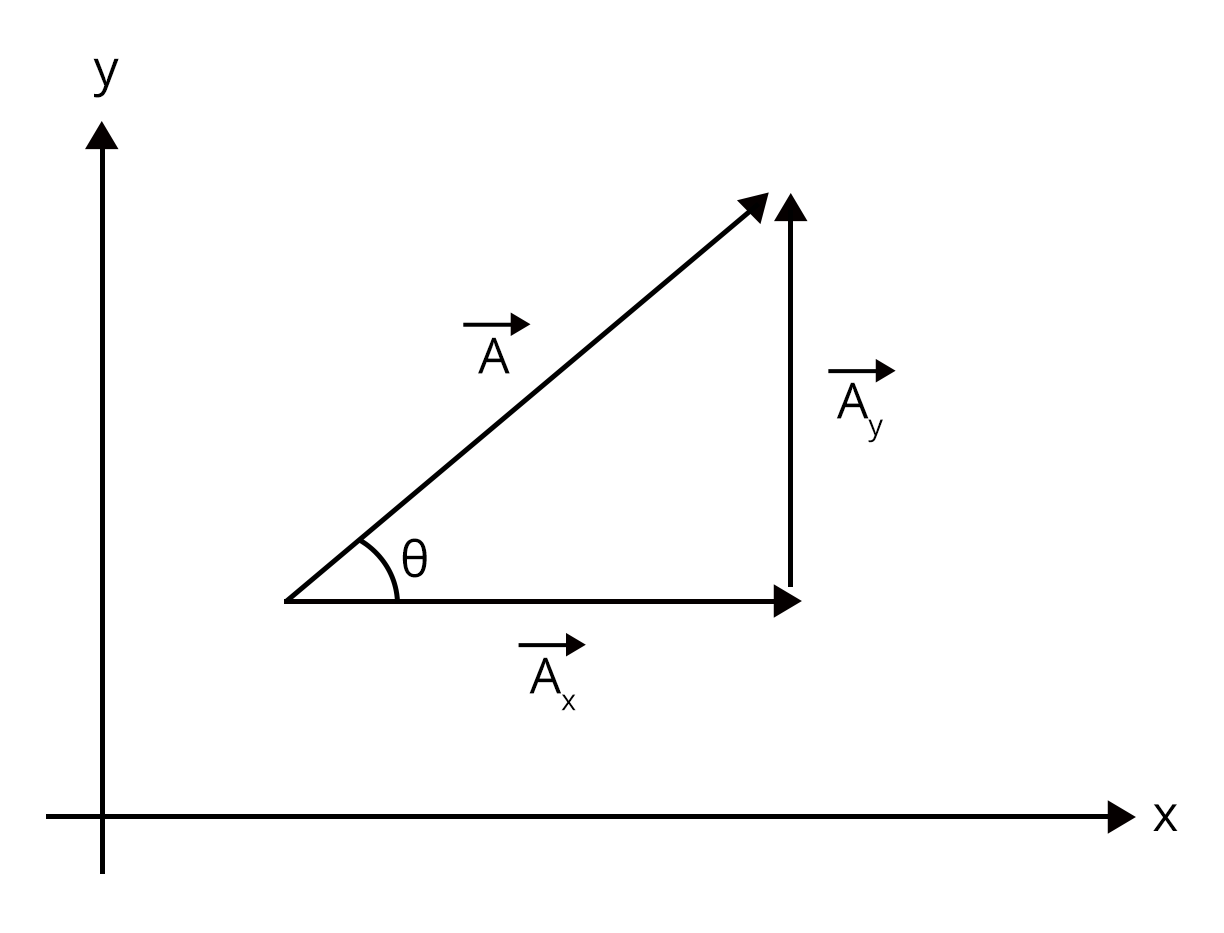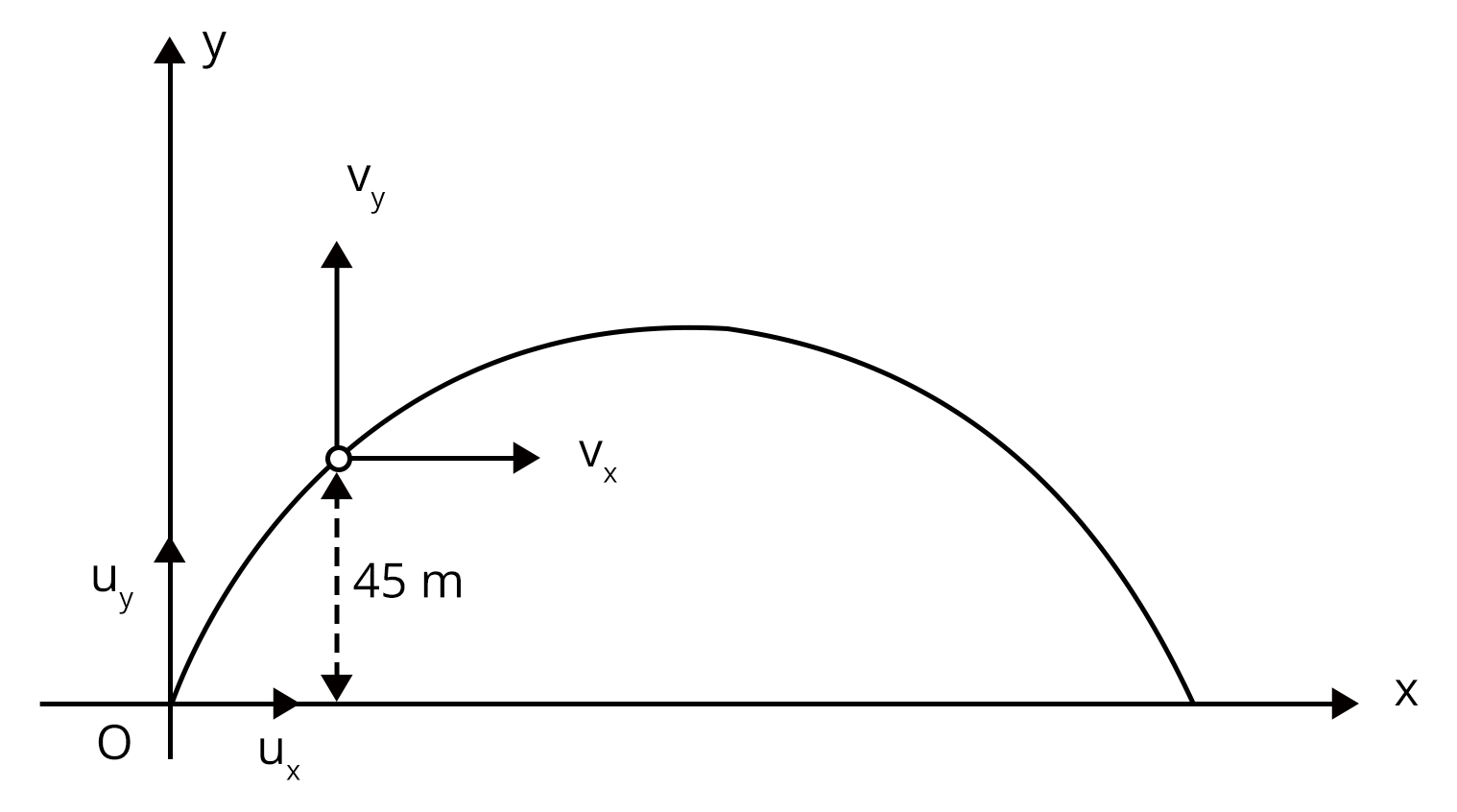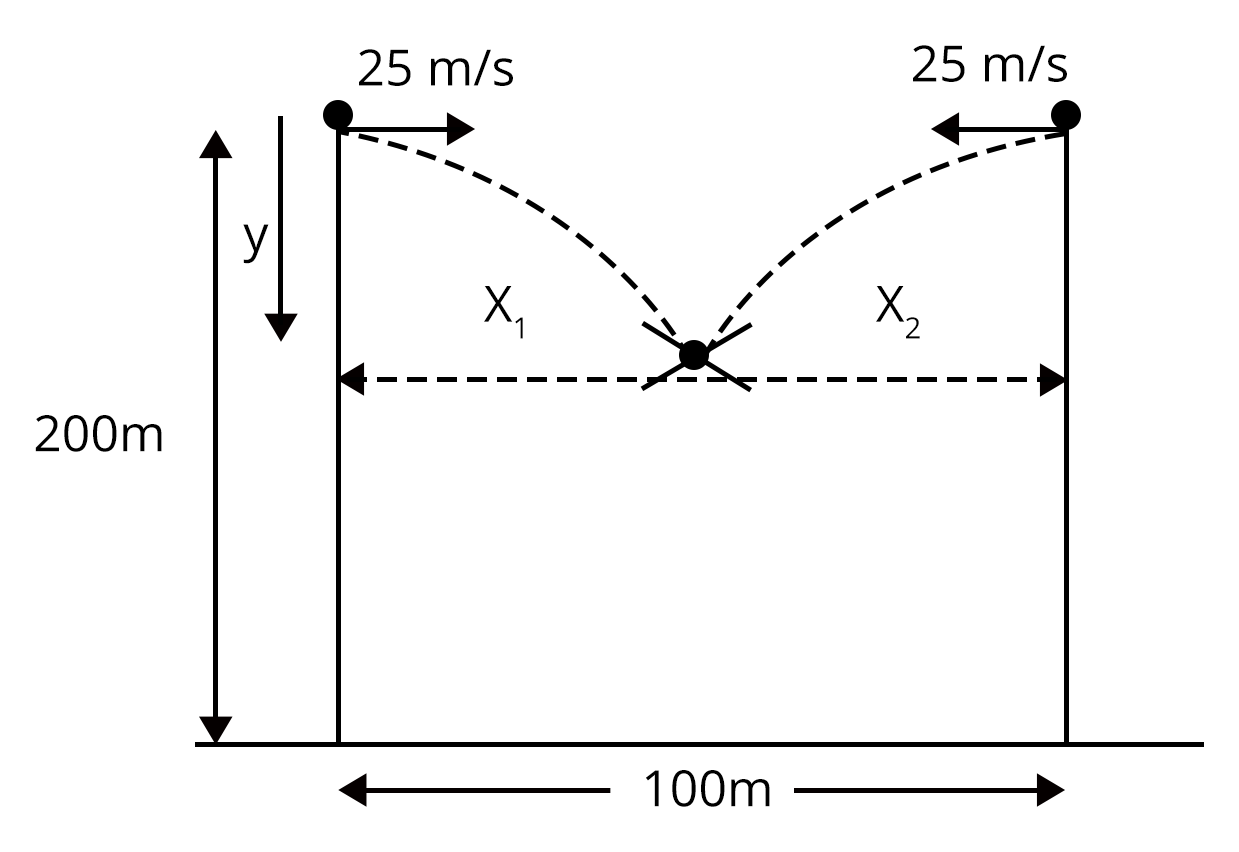Courses
Courses for Kids
Free study material
Free LIVE classes
More

# NEET Important Chapter - Motion in a Plane

Get plus subscription and access unlimited live and recorded courses## Motion In A Plane - An Important Concept For NEET

Last updated date: 19th Sep 2023
Total views: 140.7k
Views today: 4.39k

Let us first understand, what is motion in a plane? Motion in a plane means the motion of the body in two dimensions, i.e., a plane. In this chapter, we will establish concepts for position, displacement, velocity, and acceleration that are needed to describe the motion in a plane.

In the last chapter, Motion in a Straight Line, the concepts were developed for straight-line motion where directional aspects can be taken care of by using + and - signs. The discussion about motion in a plane is much more complicated because in this we have to define directional terms in two different axes, namely, the x and y-axes. So, we need to use vectors to describe motion in two dimensions. Going further in this chapter, we will learn about vectors and how to apply mathematical operators like addition, subtraction, and multiplication on vector quantities.

In this chapter, students will also learn about relative velocity in two dimensions, projectile motion, and circular motion by using vectors. Now, let us move on to the important concepts and formulae related to the NEET exam along with a few solved examples.

### Important Topics of Motion in a Plane

• Vectors

• Addition and Subtraction of Vectors.

• Resolution of Vectors.

• Motion in a plane with constant acceleration.

• Relative Velocity in Two dimensions.

• Projectile Motion.

• Projectile motion in horizontal direction from a height.

• Projectile Motion on an inclined plane.

• Uniform Circular Motion.

### Important Concepts of Motion in a Plane

 Concepts Key Points of Concepts Scalar and Vectors Scalars are the physical quantities that can be completely described by their magnitude only. For example, mass, energy, speed, and distance. Vectors are the physical quantities that need their magnitude and direction, both to be defined completely. For example, velocity, displacement, and force. Triangle Law of Vector Addition Triangle law of vector Addition states that if two non-zero vectors are represented by the sides of the triangle, taken in the same order then the resultant of those two vectors is given by the closing side of the triangle taken in the opposite order. If the two non zero vectors are A and B then, Resultant, R is given by:R = $\sqrt{A^{2}+B^{2}+2AB\cos\theta}$If resultant R makes an $angle \alpha$ with the A vector the, $\tan\alpha = \dfrac{B\sin\theta}{A+B\cos\theta}$ Resolution of a Vectors Resolution of a vector means splitting one vector into two or more vectors in different directions. Consider a vector A on the x-y plane, making an angle \theta with the x-axis as shown in the figure:Here, $A_{x} = A\cos\theta$ (along x- axis) And, $A_{y} = A\sin\theta$ (along y- axis) Relative Velocity in Two Dimensions Relative velocity is the velocity of an object with respect to the other object. Consider the two objects A and B moving with velocity $v_{a}$ and $v_{b}$ then, the relative velocity of A w.r.t. B (v_{a,b}) is given by:$v_{a,b} = v_{a} - v_{b}$ Projectile Motion Projectile motion once projected in a given direction and with a given velocity, moves under the effect of gravity only.  The locus of projectile motion is Parabola.Equation of trajectory of projectile motion is given by:$y = x\tan\theta- \dfrac{g}{2u^{2}\cos^{2}\theta}x^{2}$Maximum height reached is given by: $h = \dfrac{u^{2}\sin^{2}\theta}{2g}$Horizontal range is given by: $R = \dfrac{u^{2}\sin2\theta}{g}$ Uniform Circular Motion When the object moves in a circular motion about a fixed point with constant speed then the motion is known as Uniform Circular Motion.The speed is constant throughout in Uniform Circular Motion but the direction of speed keeps on changing. Due this change in direction, acceleration occurs which is known as centripetal acceleration $(a_{c})$ which is given by:$a_{c}=\omega^{2}R$, where, \omega is angular velocity and R is the radius of circular path.

### List of Important Formulae

 SI. No Name of the Concept Formula Resultant of two vectors R = $\sqrt{A^{2}+B^{2}+2AB\cos\theta}$ Angle made by the resultant of the vector $\tan\alpha = \dfrac{B\sin\theta}{A+B\cos\theta}$ Relative velocity in two dimensions $\vec{v_{a,b}}= \vec{v_{a}}- \vec{v_{b}}$ Equation of trajectory in Projectile Motion y = $x\tan\theta- \dfrac{g}{2u^{2}\cos^{2}\theta}x^{2}$ Maximum height reached by projectile $h = \dfrac{u^{2}\sin^{2}\theta}{2g}$ Horizontal range of the Projectile R = $\dfrac{u^{2}\sin2\theta}{g}$ Note: Horizontal range is maximum when the angle of projection is 45 degrees. 7. Centripetal acceleration $a_{c}=\omega^{2}R$

### Solved Examples

1. Two Vectors have magnitudes 6 units and 8 units respectively. What should be the angle between the vectors if the magnitude of resultant is:

1. 14 units

2. 10 units

3. 2 units

Sol:

Let,

Magnitude of A vector = 6 units,

Magnitude of B vector = 8 units,

Resultant of A and B = R,

(a) The Resultant (R) of A and B is given as 14 units. Let the angle between A and B is $angle \theta$. Then,

R = $\sqrt{A^{2}+B^{2}+2AB\cos\theta}$

14 = $\sqrt{6^{2}+8^{2}+2\times6\times8\cos\theta}$

Putting squares on both the sides, we get,

196 = $36+64+96\cos\theta$

196 = $100+96\cos\theta$

$\cos\theta$ = 1

$\theta$ = 0°

This means that the vectors A and B are parallel vectors.

(b) Now, the Resultant (R) of A and B is given as 10 units. Let the angle between A and B is $angle \theta$. Then,

R = $\sqrt{A^{2}+B^{2}+2AB\cos\theta}$

10 = $\sqrt{6^{2}+8^{2}+2\times6\times8\cos\theta}$

Putting squares on both the sides, we get,

100 = $36+64+96\cos\theta$

100 = $100+96\cos\theta$

$\cos\theta$ = 0

$\theta$ = 90°

This means that the vectors A and B are perpendicular vectors.

(c) Now, the Resultant (R) of A and B is given as 2 units. Let the angle between A and B is $angle \theta$. Then,

R = $\sqrt{A^{2}+B^{2}+2AB\cos\theta}$

2 = $\sqrt{6^{2}+8^{2}+2\times6\times8\cos\theta}$

Putting squares on both the sides, we get,

4 = $36+64+96\cos\theta$

4 = $100+96\cos\theta$

-96 = $96\cos\theta$

$\cos\theta$ = -1

$\theta$ = 180°

This means that the vectors A and B are antiparallel vectors.

Key point: Maximum value of resultant occurs when vectors are parallel and minimum value of resultant occurs when vectors are antiparallel.

2. At a height 45 m from the ground velocity of the projectile is, v = $(30\hat{i} +40\hat{j})$ m/s. Find the initial velocity of the projectile.

Sol:

Given,

• $v_{x} = 30 m/s$,

• $v_{y} = 40 m/s$,

Now, given below is the pictorial representation of the given situation:As there is no acceleration along x- direction, then velocity along x- direction will not change. therefore,  $v_{x} = u_{x} = 30 m/s$.

Now, the vertical component of the velocity will decrease as it reaches the height of 45m as the acceleration due to gravity is always in the downward direction. Using kinematic equations of motion,

$v_{y}^{2} = u_{y}^{2}-2gy$

$30^{2} = u_{y}^{2}-2\times10\times45$

$u_{y} = 50 m/s$

So, the initial velocity, u will be given by:

$u = \sqrt{u_{x}^{2}+u_{y}^{2}}$

$u = \sqrt{30^{2}+50^{2}}$

$u = 10\sqrt{34}$

Hence, the initial velocity is $10\sqrt{34}$

Key Point: Always remember that during projectile motion, horizontal velocity doesn’t change because there is no acceleration in horizontal direction.

### Previous Year Questions from NEET Paper

1. If the magnitude of sum of two vectors is equal to the magnitude of difference of two vectors, the angle between these vectors is (NEET 2016)

1. 90°

2. 45°

3. 180°

4. 0°

Sol:

Let the two vectors be A and B. It is given in the question that the magnitude of sum of the two vectors is equal to the magnitude of the difference between the two vectors. Therefore,

$|A+B\lvert=|A-B\lvert$

$\sqrt{A^{2}+B^{2}+2AB\cos\theta}$ = $\sqrt{A^{2}+B^{2}-2AB\cos\theta}$

$4AB\cos\theta = 0$

$\theta = 90°$

Hence, the correct answer is option (a)

Trick: Magnitude of sum of two vectors is always equal to difference of two vectors when the two vectors are perpendicular.

1. Two bullets are fired simultaneously towards each other from the rooftops of two buildings 100 m apart and of the same height, 200 m with the same velocity of 25 m/s. When and where will the bullets collide (NEET 2019)

1. At 2s at height of 180 m

2. At 2s at a height of 20 m

3. At 4s at a height of 120 m

4. They will not collide

Sol.

Let the bullets collide at time, t and a point whose distance from one building is x1 and other building is x2, as shown in figure,Therefore,

x1 + x2 = 100 m,

Also,

x1 = ut = 25t,

x2 = ut = 25t,

Then,

x1 + x2 = 100 m

25t + 25t = 100m,

50t = 100,

t = 2s,

Now, the vertical distance is given by,

y = $\dfrac{1}{2}gt^{2}$

y = $\dfrac{1}{2}\times10\times 2^{2}$

y = 20 m

So, the height from the ground will be (200m - 20m) = 180 m

Therefore, the bodies will collide at time 2 secs at the distance of 180 m from the ground. Hence, option (a) is the correct answer.

Trick: In any type of case, when the two particles are projected simultaneously, they will meet at the same time and position.

### Practice Questions

• A particle is thrown over a triangle from one end of a horizontal base and after grazing the vertex falls on the other end of the base. If $\alpha$ and $\beta$ be the base angles and $\theta$ the angle of projection, prove that $\tan\theta= \tan\alpha+\tan\beta$

• The resultant of two forces, one double the other in magnitude, is perpendicular to the smaller of the two forces. Then what is the angle between the two forces? (Ans. 120°)

### Conclusion

In this article we have provided important information regarding the chapter motion in a plane such as important concepts, formulae, etc.. Students should work on more solved examples for securing good grades in the NEET exam.

See More## NEET Important Dates

View All Dates
NEET 2023 exam date and revised schedule have been announced by the NTA. NEET 2023 will now be conducted on 7-May-2023, and the exam registration closes on last week of Dec 2022. You can check the complete schedule on our site. Furthermore, you can check NEET 2023 dates for application, admit card, exam, answer key, result, counselling, etc along with other relevant information.
See More
View All Dates## NEET Information

Application Form
Eligibility Criteria
Reservation Policy
NTA has announced the NEET 2023 application form release date on the official website https://neet.nta.nic.in/. NEET 2023 Application Form is available on the official website for online registration. Besides NEET 2023 application form release date, learn about the application process, steps to fill the form, how to submit, exam date sheet etc online. Check our website for more details.## NEET 2023 Study Material

View NEET Syllabus in Detail
View NEET Syllabus in Detail## NEET 2023 Study Material

View all study material for NEET
All
Physics
Chemistry
Biology
See All## NEET Question PapersView all NEET Important Books
Biology
NCERT Book for Class 12 Biology
Physics
NCERT Book for Class 12 Physics
Chemistry
NCERT Book for Class 12 Chemistry
Physics
H. C. Verma Solutions
See All## NEET Mock Tests

View all mock tests
NEET 2023 free online mock test series for exam preparation are available on the Vedantu website for free download. Practising these mock test papers of Physics, Chemistry and Biology prepared by expert teachers at Vedantu will help you to boost your confidence to face the NEET 2023 examination without any worries. The NEET test series for Physics, Chemistry and Biology that is based on the latest syllabus of NEET and also the Previous Year Question Papers.
See More## NEET 2023 Cut Off

NEET Cut Off
NTA is responsible for the release of the NEET 2023 cut off score. The qualifying percentile score might remain the same for different categories. According to the latest trends, the expected cut off mark for NEET 2023 is 50% for general category candidates, 45% for physically challenged candidates, and 40% for candidates from reserved categories. For the general category, NEET qualifying marks for 2022 ranged from 715-117 general category, while for OBC/SC/ST categories, they ranged from 116-93 for OBC, 116-93 for SC and 116-93 for ST category.
See More## NEET 2023 Results

The NEET 2023 result will be published by NTA on https://neet.nta.nic.in/ in the form of a scorecard. The scorecard will include the roll number, application number, candidate's personal details, and the percentile, marks, and rank of the candidate. Only those candidates who achieve the NEET cut-off will be considered qualified for the exam.
See More
Rank List
Counselling
Cutoff
NEET 2023 state rank lists will be released by the state counselling committees for admissions to the {state-quota-percentage} state quota and to all seats in private medical and dental colleges. NEET 2023 state rank lists are based on the marks obtained in entrance exams. Candidates can check the NEET 2023 state rank list on the official website or on our site.## NEET Top Colleges

View all NEET 2023 Top Colleges
Want to know which Engineering colleges in India accept the NEET 2023 scores for admission to Engineering? Find the list of Engineering colleges accepting NEET scores in India, compiled by Vedantu. There are 1622 Colleges that are accepting NEET. Also find more details on Fees, Ranking, Admission, and Placement.
See More## FAQs on NEET Important Chapter - Motion in a Plane

FAQ

1. What is the weightage of the motion in a plane in NEET?

Nearly 2-3 questions are expected in the exam from this chapter covering about 10 marks which makes about 2% of the total marks. Students should concentrate more on the important topics of motion in a plane like projectile motion and relative motion in two dimensions. These motion in a plane notes are written in such a way that all the important points are covered for students in a concise manner for quick revision.

2. What are the key points that need to be practiced for solving questions from motion in a plane?

Students should practice understanding vectors for solving the questions from motion in a plane. Also, understand the relationship between different variables in the equations of motion. This will help students to solve any kind of question. The table for motion in a plane formula should be kept in mind.

3. Are previous year questions enough for NEET?

To score 640+ in NEET, learning and revising NCERT (both 11th and 12th), and previous year NEET papers are sufficient. Solving previous 10-year NEET examinations offers us a tremendous advantage because 6-7 questions with identical alternatives are guaranteed to be repeated every year.## Notice board

NEET News
NEET Blogs